Back

## Sweet Sixteen

Given the integers $1$ through $9$ in a $3 \times 3$ grid, we can rearrange those integers so that every row and column has a sum of $15:$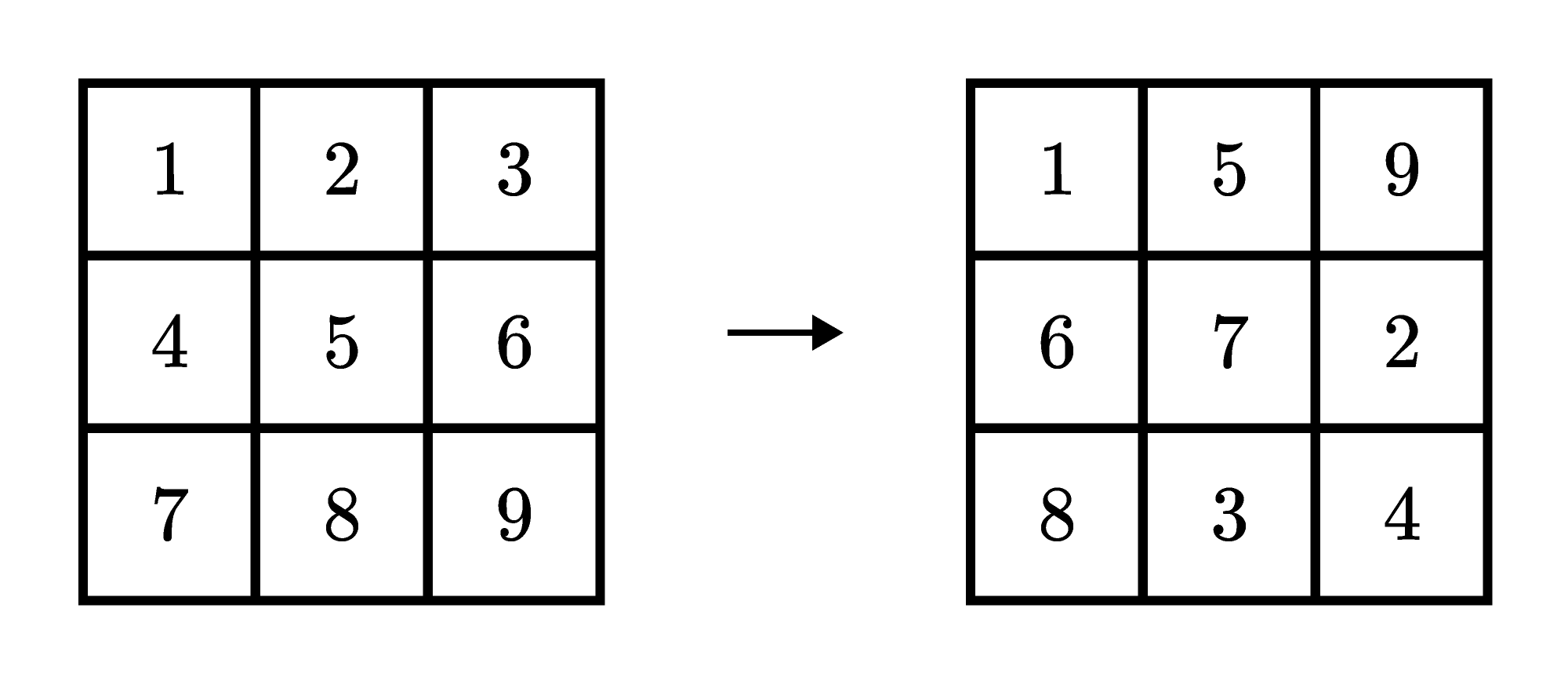This is just one example; there are more arrangements that work.

Suppose that, instead, starting with that same initial arrangement of $1$ through $9$ in a $3 \times 3$ grid, we replace any number of the integers with $0$ to try to make every row and column have the same sum.

This problem has no solution $($other than the trivial solution of replacing every integer with $0).$ To see why — and why for a problem like today’s challenge — keep reading. Otherwise, jump ahead to the challenge.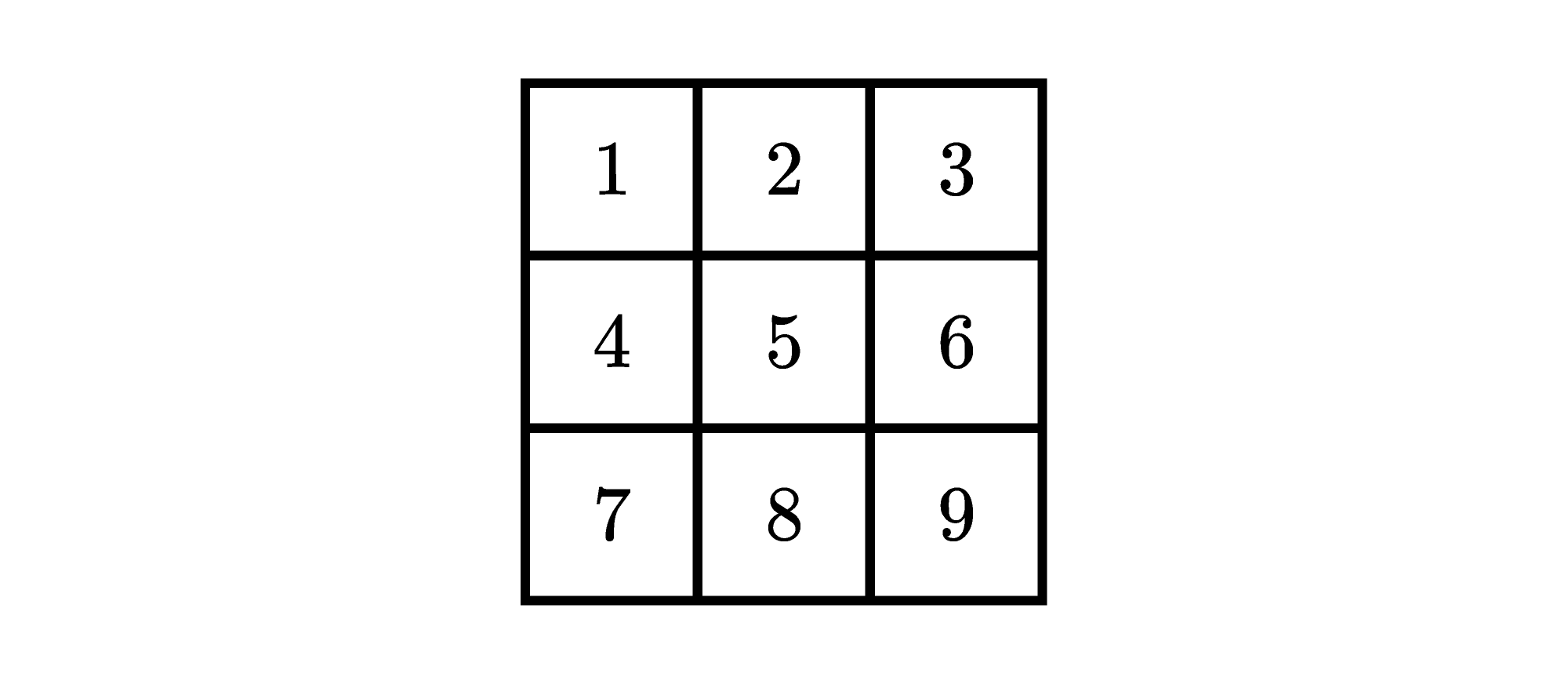As arranged, the sum in the top row of the grid is $6.$ To make all the rows and columns have the same sum by replacing integers with $0$s, each row and column would need to have a sum of $6$ or less, to match that top row. Looking at the bottom row, the only way to make it have a sum of $6$ or less is by making it have a sum of $0.$ Then every integer in the grid would have to be replaced with $0.$

In today’s challenge, the starting $5 \times 5$ grid is filled with integers, but they aren’t $1$ through $25$ in order and some of the integers repeat. Here we’ll work through a similar example with a $4 \times 4$ grid: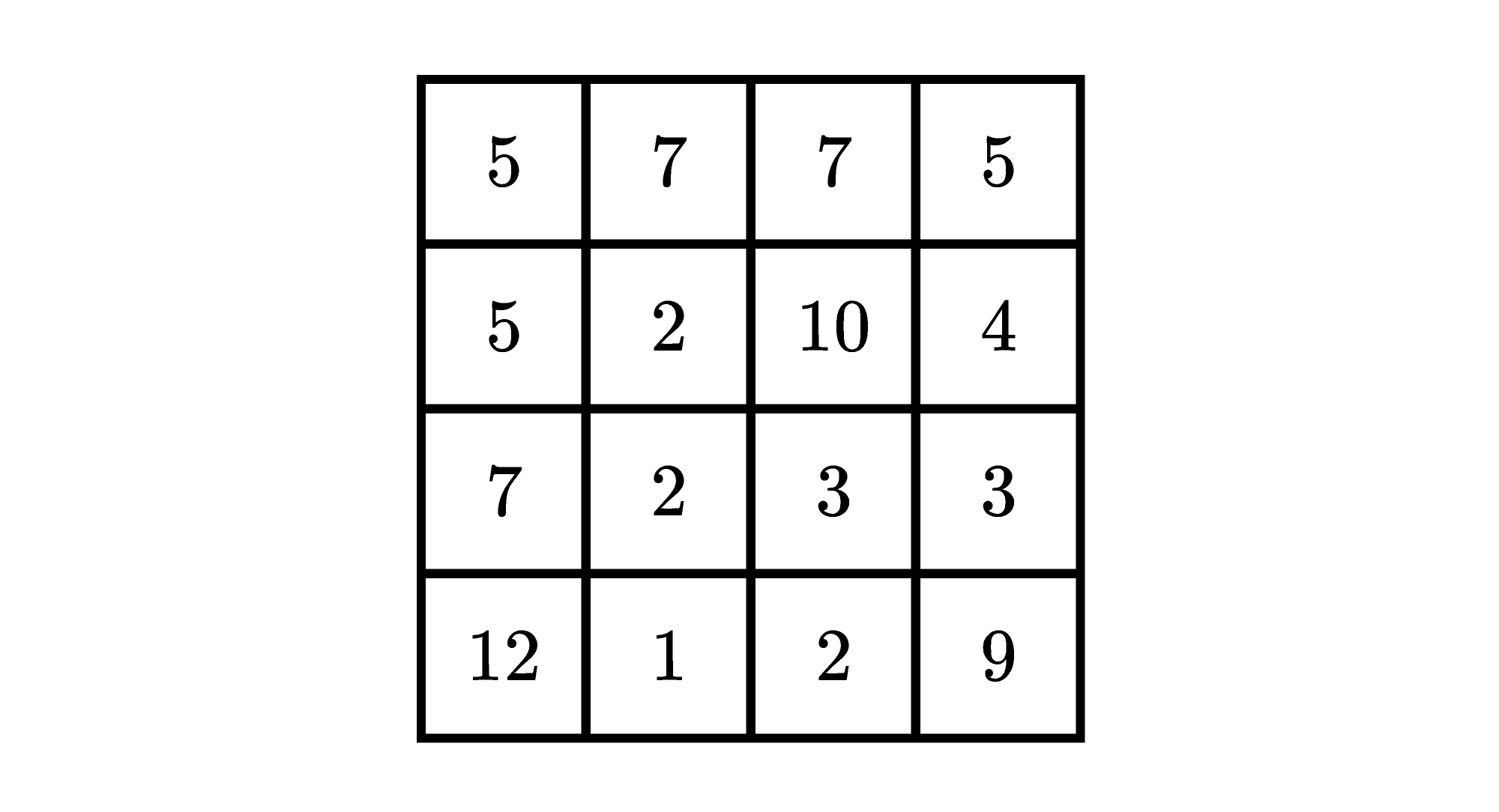Given this grid, the goal is to replace any of the integers with $0$ so that the sum of every row and column is $12.$

The most challenging part can be deciding where to begin. Try to find the most restricted part of the grid where a row or column can only sum to $12$ in one or two ways. Consider the second row: the only way to make a sum of $12$ with $5, 2, 10,$ and $4$ is $2+10.$ So the $5$ and the $4$ need to be replaced with $0$s: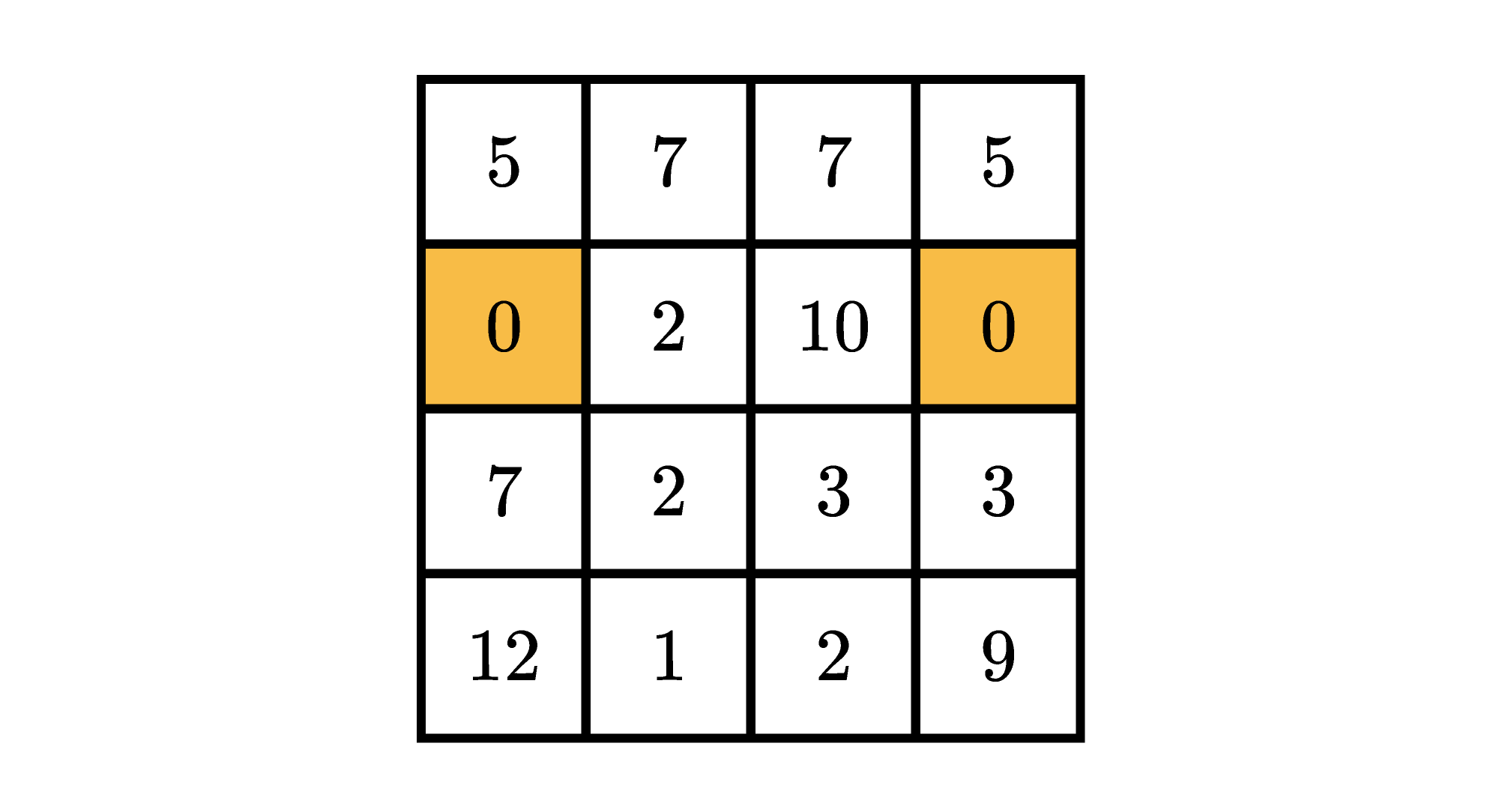Now we know that the $10$ and $2$ in the second row cannot be replaced with $0$s. To make the third column with that $10$ in it sum to $12,$ we must replace the $7$ and $3$ with $0$s: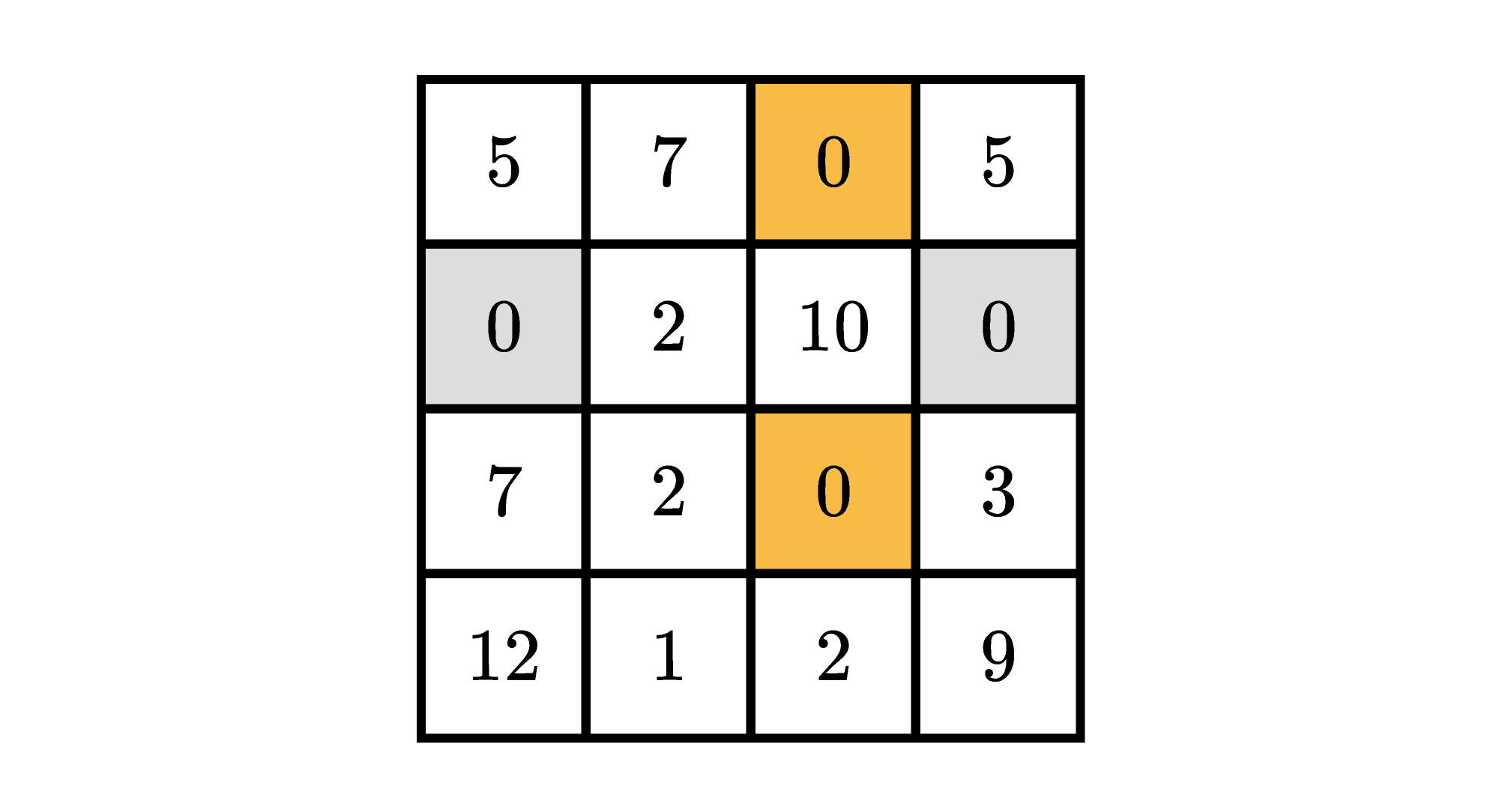In the second column, we need each one of $7, 2, 2,$ and $1$ to sum to $12.$ So none of those get replaced with $0$s. In particular, the bottom row must use that $1$ in its sum. Then we must also use the $2$ and $9$ in the bottom row, while the $12$ must be replaced with a $0.$ Meanwhile, in the top row, one of the $5$s must be replaced with a $0.$ The $5$ in the left column we need, the $5$ in the right column we don’t need.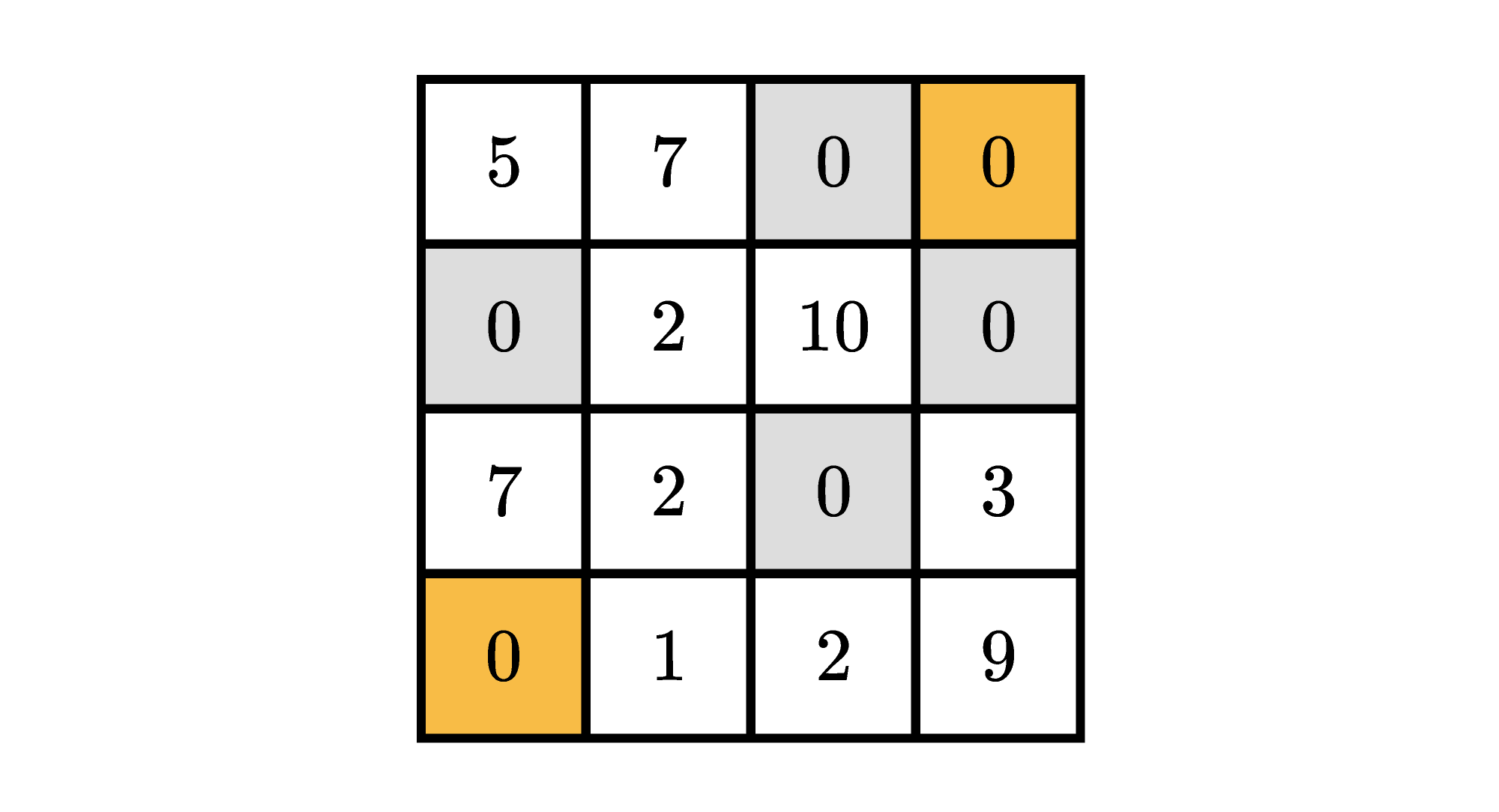By replacing six of the numbers with $0$s, we now have every row and column sum to $12.$ Can you do the same with a slightly larger square and target sum?

# Today's Challenge

In this challenge, you can replace any number in the grid below with a $0:$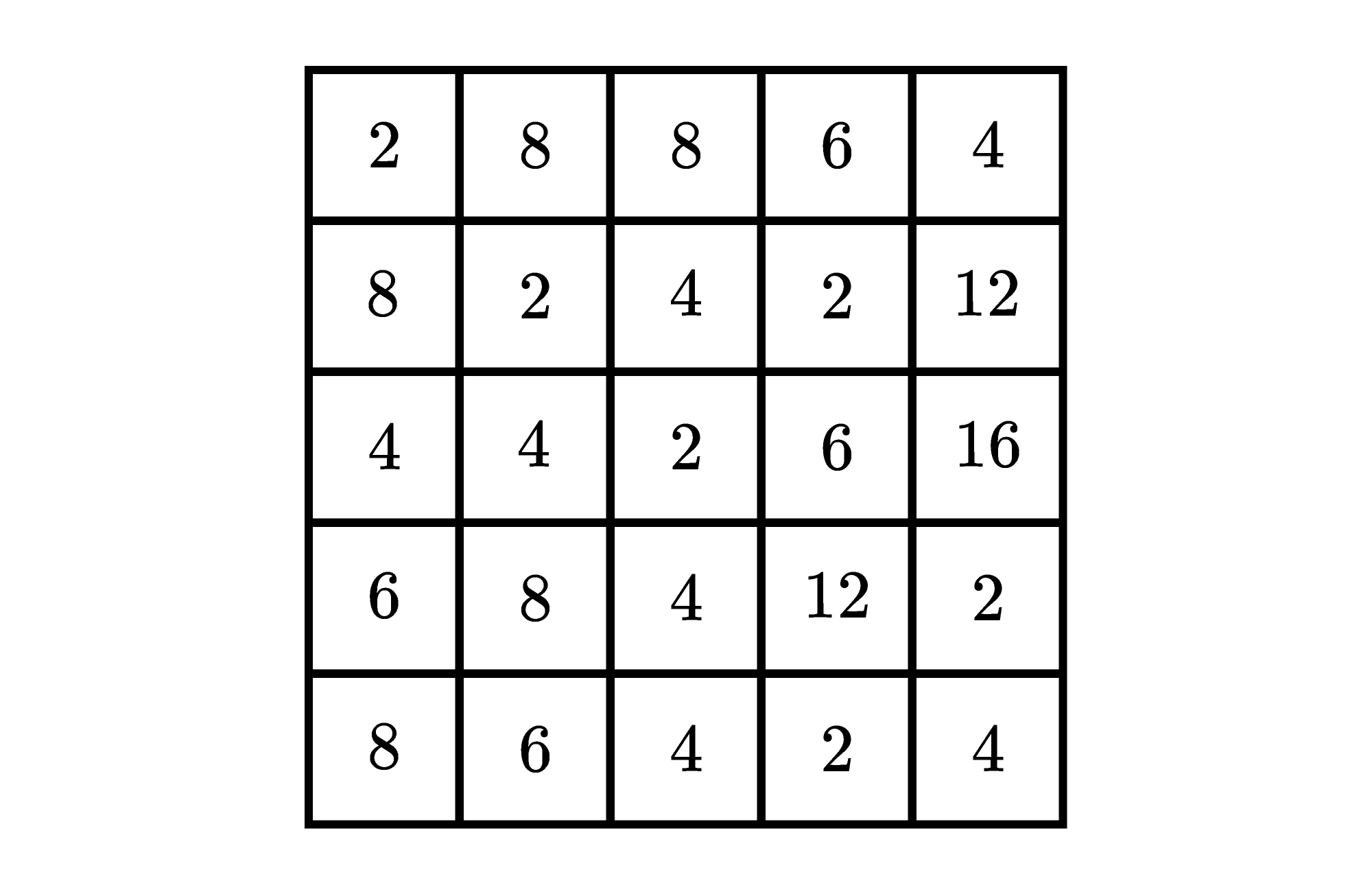How many numbers in the grid must be replaced with $0$s to make the sum of every row and column $16?$We are sunsetting our community features by July 2, 2021. Learn more here.
×

Problem Loading...

Note Loading...

Set Loading...